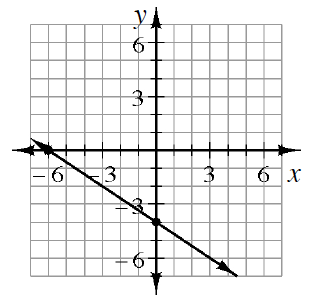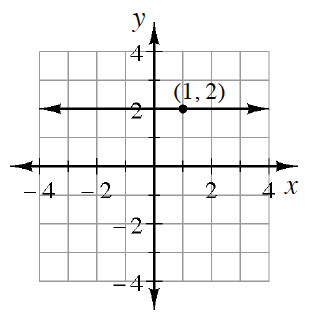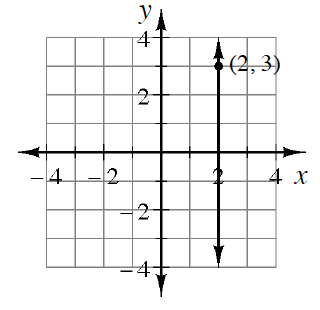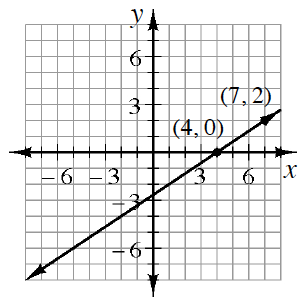### Home > CCA2 > Chapter 2 > Lesson 2.1.1 > Problem2-9

2-9.

Write the equation for each graph.

1.What is the $y$-intercept? Pick two points and determine the slope.

$y = - \frac { 2 } { 3 } x - 4$

1.Refer to part (a).

1.Which value ($x$ or $y$) is equal to $2$ on this line? (Look at the point.)

All vertical lines have undefined slope and an equation of the form $x=k$.

1.Refer to part (a).